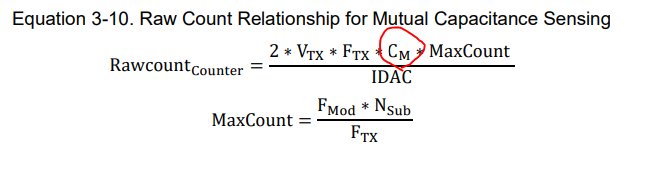cancel
Showing results for
Did you mean:

PSoC™ 4Contributor

Regarding how to check CSX mutual capacitance.

Hello Cypress.

Is there API for calculating mutual capacitance(Cm)?

I think that CapSense_GetSensorCapacitance only calculates TX capacitance and RX capacitance separately.

CSD can calculate self capacitance(Cp).

It was possible to judge whether device could drive the sensor by checking obtained value.

If user uses CSX, how should they check mutual capacitance whether Cm is smaller than maximum value specified by device?

Below is calculation formula of CSX RawCount.

Cm is used in the CSX RawCount formula.

So it is necessary to know Cm in advance in order for CSX to output RawCount.

How does CSX calculate Cm before calculating RawCount?Best Regards.

Yutaka Matsubara.

1 SolutionModerator

Re: Regarding how to check CSX mutual capacitance.

Hello YuMa_1534086

We don't have an API that directly gives the mutual capacitance value. Please refer this KBA Mutual Capacitance Calculation of a CapSense Sensor Through Firmware - KBA222425 for details on how to calculate Cm for your design.

Thanks and regards

Hari

8 RepliesModerator

Re: Regarding how to check CSX mutual capacitance.

Hello YuMa_1534086

We don't have an API that directly gives the mutual capacitance value. Please refer this KBA Mutual Capacitance Calculation of a CapSense Sensor Through Firmware - KBA222425 for details on how to calculate Cm for your design.

Thanks and regards

HariContributor

Re: Regarding how to check CSX mutual capacitance.

Hari-san.

Q1)

In KBA222425, Cm is calculated based on RawCount is outputted from PSoC4.

But I think that PSoC4 should have calculated Cm before calculating RawCount.

Because Cm value is included in CSX RawCount formula.

How does PSoC4 calculate Cm internally before calculating RawCount?

Q2)

Does Cypress have any plans to add API to calculate Cm directly in the future?

Unlike CSD, CSX cannot calculate Cm directly, so it is difficult to judge whether PSoC can drive sensors.

Best Regards.

Yutaka MatsubaraModerator

Re: Regarding how to check CSX mutual capacitance.

Hello Yutaka-san

1. Yes. Cm is a physical parameter which dictates the value of raw-counts. PSoC does not calculate raw counts with value of Cm, rather, the value of Cm determines the raw counts. When Cm increases, raw counts also increase and when Cm decreases, raw counts decrease.

2. We have forwarded this to our internal team who will look into this request. Thank you for the suggestion and your interest in Cypress products.

Please note that driving the sensors still depend on parasitic capacitance (Cp) of the Tx electrode and not on the mutual capacitance formed (Cm).

Thanks and regards

HariContributor

Re: Regarding how to check CSX mutual capacitance.

Hari-san.

Does PSoC not calculate Cm internally?

If PSoC does not calculate Cm, I think that CSX RawCount formula in design guide is wrong.

Because RawCountCounter formula contains Cm parameter.

And CSX RawCount formula is “MaxCount – RawCountCounter".

So if design guide formula is correct, PSoC should calculate Cm value at first.

Because PSoC can not calculate CSX RawCount if PSoC can not calculate Cm.

Is CSX RawCount formula in design guide wrong?

Or if formula is correct, how does PSoC4 calculate Cm internally before calculating RawCount?

Best Regards.

Yutaka MatsubaraModerator

Re: Regarding how to check CSX mutual capacitance.

Hello Yutaka-san,

The formula given in design guide is a mathematical representation of the physical parameter's calculation. The CapSense component in the controller does not calculate raw counts using this formula. Rather, there is an electrical circuit that converts the physical parameter (Cm) into digital quantity (raw counts).

In CSX design, there is a Tx electrode and an Rx electrode and a mutual capacitance is formed between them (Cm). The Tx electrode is switched with a square wave that switches between VDDIO and ground. There are two switches, SW1 and SW2 which connect to integrating capacitors. During one half cycle, the voltage on CintA increases above Vref. IDAC is configured in sinking mode which brings the voltage back to Vref and during this time, the counter is ON. During the next cycle, CintB is connected and voltage drops below Vref (since Tx is LOW). IDAC is in sourcing mode and brings the voltage back to Vref. Again, the counter is ON during the time in which the CintB is not Vref.

This happens for a number of cycles (called number of sub-conversions and is set in the component) and the sum of all sub-conversions is the raw counts that we observe.This raw counts is indeed based on the mutual capacitance formed by the equation given, but the equation is not the way we obtain the raw counts in the controller. The equation provides the mathematical approximation for the raw counts that is expected and is provided in the design guide to help in tuning the sensors.

You can refer CapSense Design guide for more details on CSX operation.

Thanks and regards

HariContributor

Re: Regarding how to check CSX mutual capacitance.

Hari-san.

Thank you for response.

>>> The CapSense component in the controller does not calculate raw counts using this formula.

Same as CSX, does not CSD use below formula when CSD calculates RawCount?CSD has API for calculate Cp value.

Does this API just calculate Cp by use above formula?

Best Regards.

Yutaka MatsubaraModerator

Re: Regarding how to check CSX mutual capacitance.

Hi Yutaka-san

Yes. That is correct. CSD also has hardware component that measures the raw counts.

The API just back calculates Cp from the equation since raw counts is already present. The method of calculation is like the KBA that was shared (since all other parameters are known to the controller, Cp can be calculated).

Best regards

HariContributor

Re: Regarding how to check CSX mutual capacitance.